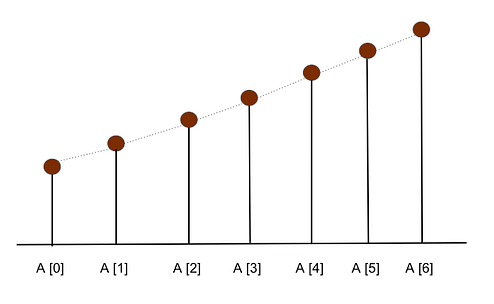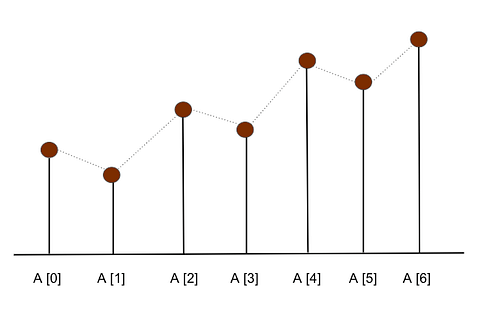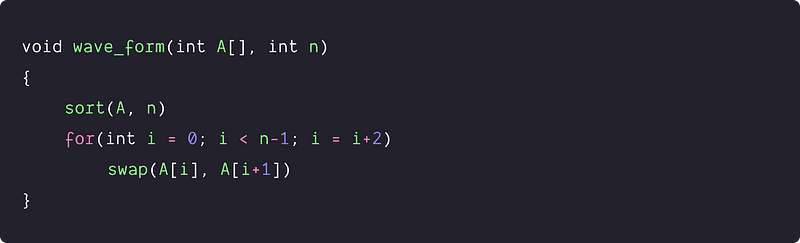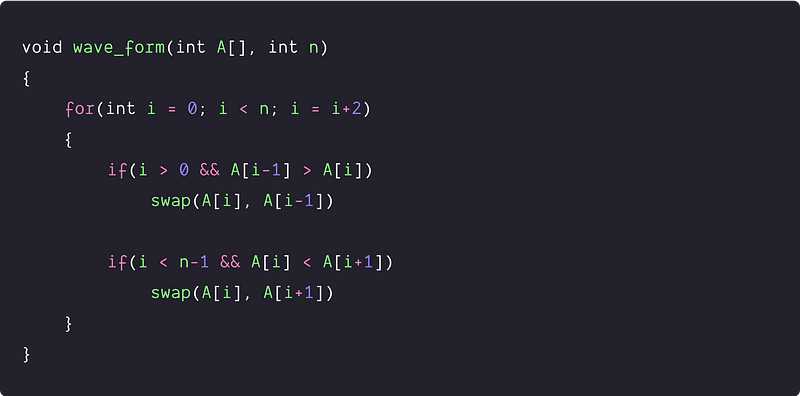# Sort an array in a waveform

Key takeaway: A good problem to learn the problem solving using sorting and single scan (Incrementing the loop by 2). Such problems are popular where we rearrange the input in place to get the final output.

### Let’s understand the problem

Given an array of integers, sort the array into a wave-like arrangement. In other words, An array A[0..n-1] is sorted in wave form if A >= A <= A >= A <= A >= ….

Note: There can be multiple possible answers but we just return any one possible wave-like arrangement.

Examples

Input: A[] = [1, 2, 3, 4]

Output: A[] = [2, 1, 4, 3] or [4, 1, 3, 2] or any other array that is in wave form

Input: A[] = [20, 10, 8, 6, 4, 2]

Output: A[] = [20, 8, 10, 4, 6, 2] or [10, 8, 20, 2, 6, 4] or any other array that is in a wave form.

### Discussed solution approaches

1. A brute force solution using sorting
2. An efficient solution using a single scan: loop increment by 2

### A brute force solution using sorting

Solution Idea

If we look at the wave-like output, the values are organized in an alternate order of max and min values. So how do we transform the given input in such order? If our input array is sorted then, can we arrange the array elements in waveform? Let’s think!

Suppose our input array is sorted, then all the elements will be arranged in the increasing order, i.e., A ≤ A ≤ A ≤ A≤ ….≤A[n-2] ≤ A[n-1]. If we pick the elements in pairs from the start and swap the adjacent elements, it gets arranged in the form of the wave order. (Think. How?)

Visualization of the Sorted ArrayVisualization after swapping adjacent elements in the sorted arraySolution PseudocodeTime and space complexity analysis

Here the time complexity is dominated by the sorting algorithm. It will be O(nlogn) if we use an efficient sorting algorithm like quicksort, merge sort, or heap sort. Time complexity = Time complexity of sorting + Time complexity of swapping the adjacent element = O(nlogn) + O(n) = O(nlogn)

Space complexity: O(1), if we use heap sort. O(n), if we use merge sort.

### An efficient solution using a single scan: Increment by 2

Solution Idea

The critical question is - can we solve this problem in O(n) time complexity, i.e., without using sorting. Let's think and explore one level further.

From the given output pattern, if we ensure that values at all the even positions are greater than the value at the odd position, then we can achieve the wave-like structure. In other words, for every even index i, we need to ensure this order in triplet A[i-1], A[i] and A[i+1] => A[i-1] < A[i] > A[i+1]

• Case 1: If A[i-1] < A[i] < A[i+1] then swap A[i] and A[i+1] to get the order A[i-1] < A[i] > A[i+1].
• Case 2: If A[i-1] > A[i] > A[i+1] then swap A[i-1] and A[i] to get the order A[i-1] < A[i] > A[i+1].
• Case 3: If A[i-1] > A[i] < A[i+1] then swap A[i-1] and A[i], we will get the order A[i-1] < A[i]. Now if A[i] < A[i+1] then we should swap A[i] and A[i+1].
• Case 4: If A[i-1] < A[i] > A[i+1] then no need to do any swapping because values are already arranged in a required order.

• If (A[i-1] > A[i]) then we should just swap A[i-1] and A[i]
• If (A[i] < A[i+1]) then we should just swap A[i] and A[i+1]

We can run a loop to compare adjacent elements at every iteration and ensure that all elements at even positions are greater than their adjacent elements.

Solution Steps

1. We run a loop incrementing by two to Iterate over even positioned elements.
2. If the current element is smaller than the last odd element, then swap the current element with the last element.

``````if(i > 0 && A[i-1] > A[i])
swap(A[i], A[i-1])
``````
3. If the current element is smaller than the next odd element, then swap the current element with the last element.

``````if(i < n-1 && A[i] < A[i+1])
swap(A[i], A[i+1])
``````

Solution PseudocodeTime and space complexity analysis

The time complexity for the above algorithm would be O(n) because we are linearly traversing the elements of the array. Space complexity: O(1) as no extra memory is being used.

### Critical ideas to think!

• How do we modify the above algorithms to output the lexicographically smallest solution?
• Does the above algorithm work if adjacent elements are equal?
• Can we pick elements at odd positions and compare them with elements at even positions?
• How many comparisons are made in the 2nd approach?
• Can we solve this problem using another approach?

### Comparisons of time and space complexities

• Brute force solution: Time = O(nlogn), Space = O(1), if we use heap sort.
• Using a single scan: Time = O(n), Space = O(1)

### Suggested coding questions to practice

Thanks to Navtosh Kumar for his contribution in creating the first version of the content. Please write comments if you find an error or you want to share more insights about the topic. Enjoy learning, Enjoy coding, Enjoy algorithms!

Get well-designed application and interview centirc content on ds-algorithms, machine learning, system design and oops. Content will be delivered weekly.